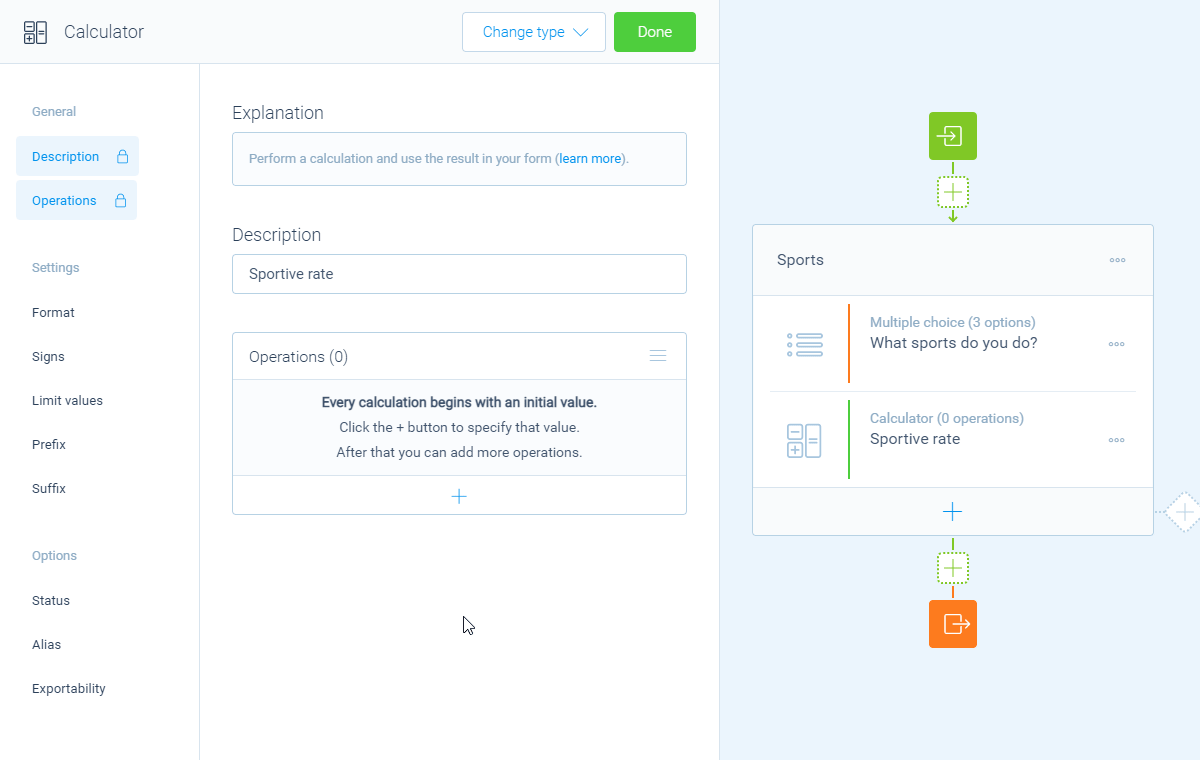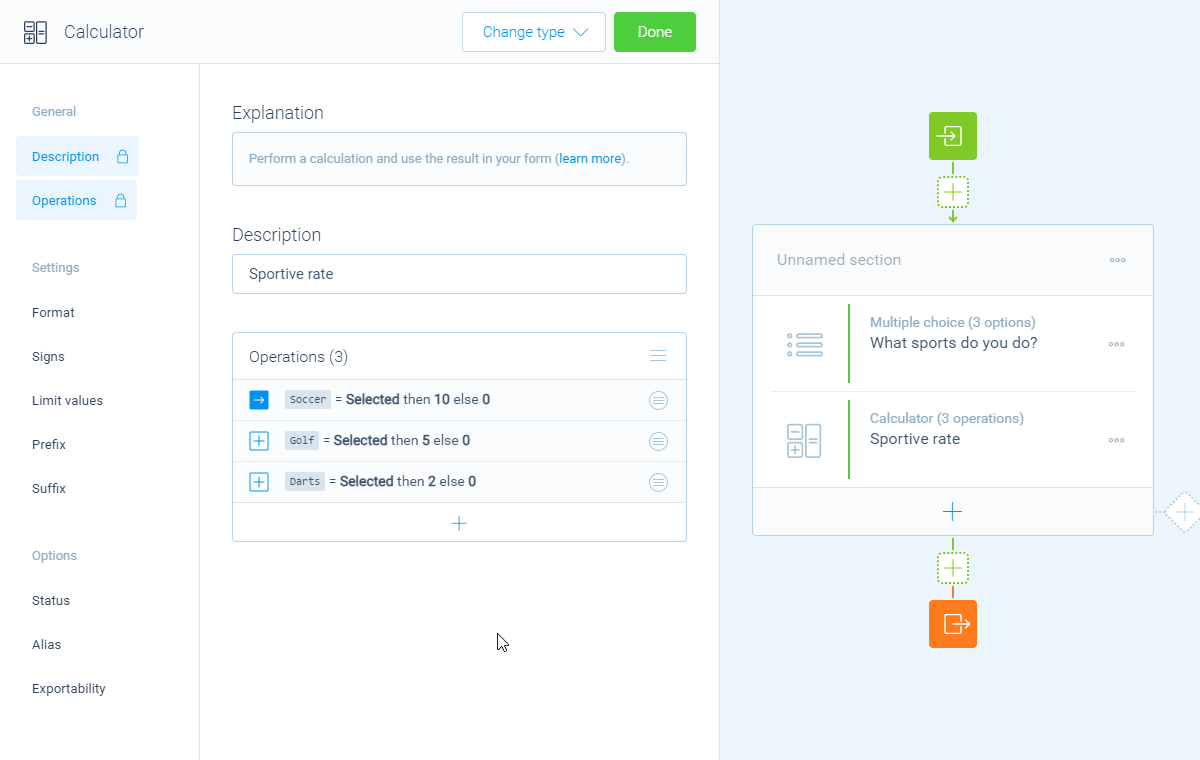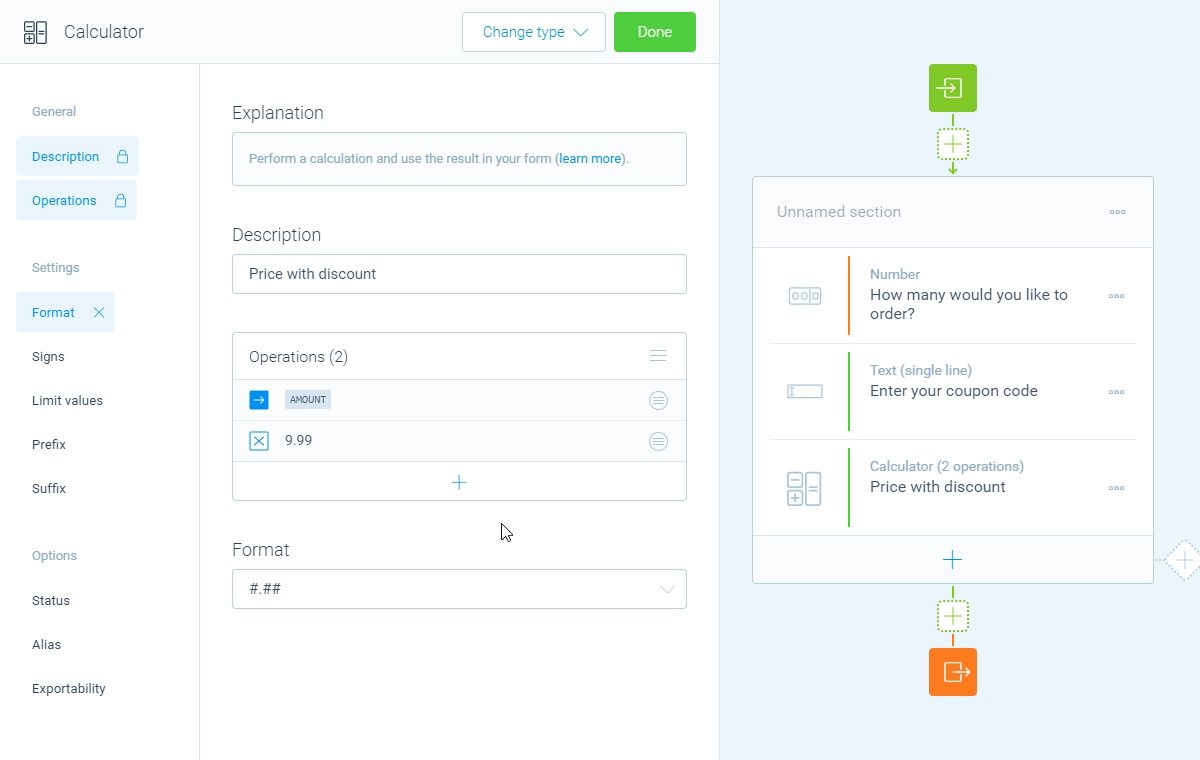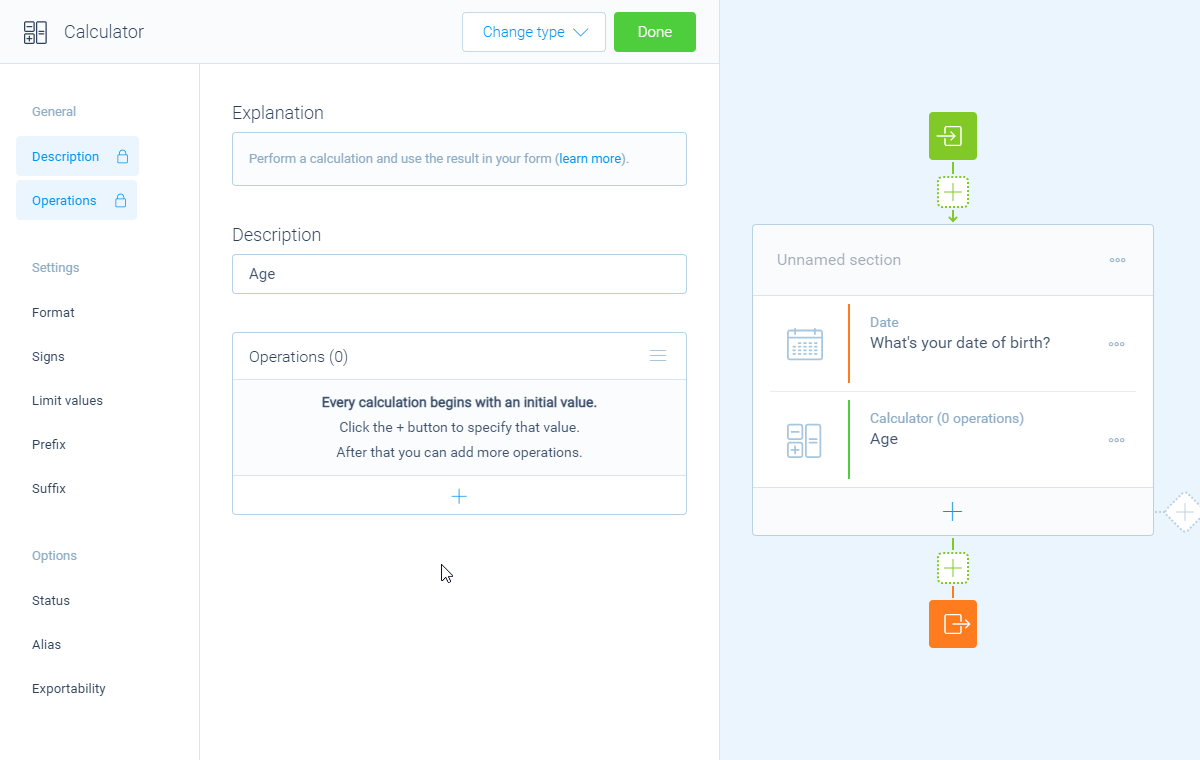Using logic5 Min.2 Min.

## When to use

Use the calculator block when you want to perform calculations inside your form. If you want to do calculations with the answers of your respondents, you can use those in realtime. For example:

• Calculate a quiz score of a respondent, based on the given answer in a picture choice question;
• Calculate a total price, based on the selected products and the entered amount of each product;
• Calculate a discount, based on the entered coupon code;
• Calculate an age of a respondent, based on the entered date of birth;
• Calculate a BMI of a respondent, based on the entered length and weight.

These are just some examples. Basically you can calculate anything you want with the calculator block. Please have a look at our calculator features overview to see everything you can do with the calculator block.

## How to use

There are several options to determine the value of each operation in your calculation. One of those options is to use the given answers of your respondents to questions in your form. This enables you to perform realtime calculations based on the input of your respondent and calculate personalized outcomes.

From the menu to add an operation, you will see a list of questions in your form that you can use to calculate with. It depends on the question type what you exactly can do with it. In general these are the options you have to use given answers:

• Recall value;
• Score selected option(s);
• Check selected option(s);
• Count selected option(s);
• Compare value;
• Age date.

We will explain each of these options below.

### Recall value

This lets you use the supplied value from your respondent inside the operation, for example from a number block, rating block or scale block.

### Score selected option(s)

This lets you score all options of question blocks where the respondent can choose from a list of options, for example a dropdown block, radio buttons block or a picture choice block (single and multiple selection). The calculator will show all the options that are available in that question block and you can enter the score that each option represents. The score of each selected item will be used as the value for the operation.

### Check selected option

This lets you check if a certain option is checked/selected by your respondent, for example from a multiple choice block (multiple selection) or a picture choice block (multiple selection). Based on the selection you can determine the desired values to use in the operation:

• A value when the option is selected;
• A value when the option is not selected.In this example we add up the values we give for each individual selected option: a value when the option is selected and a value when the option is not selected.

### Count selected options

This counts the amount of selected options by your respondent, for example from a checkboxes block, a multiple choice block (multiple selection), or a picture choice block (multiple selection).

The amount of selected items will be the value of the operation.In this example we already added up some selected values. After that we want to calculate the average of those values by dividing the last outcome with the count of the selected items.

### Compare value

This lets you compare the supplied value from your respondent to a condition that you compose. It depends on the question type which compare modes are available. Based on the comparison you can determine the desired outcome to use in the operation. There are two possible outcomes of a comparison:

• A value when the comparison is true;
• A value when the comparison is false.In this example we compare a supplied text with value DISCOUNT. If the comparison is true, a discount of \$4.99 is subtracted. Else no discount is subtracted.

### Age date

This lets you calculate the age based on a date input by your respondent, for example a date of birth that your respondent supplies. You can use several dates to calculate the age from, for example the current date, a fixed date, or another supplied date by your respondent. And you can determine how the age should be outputted: in years, months, days, or even hours, minutes, seconds or milliseconds.

The age will be used as the value for the operation.In this example we get the age of the respondent based on the supplied date of birth.

### Other usages

The above examples all show how to use given answers from your respondents as inputs to determine what should be calculated. But you can also use the given answers in other steps of calculator operations, for example inside comparisons or as output values of an operation. This makes the usage of given answers extremely powerful and flexible.

The calculator block has lots of features, so we have several ways to learn all about it.

### Help center

Our help articles help you out on all different aspects of the calculator:

### Overviews

We also made some overviews of the capabilities that the calculator block provides: